Supervised learning, unsupervised learning, parameter learning and model and cost functions.

# Introduction to Machine Learning: Supervised and Unsupervised Learning

I would like to give full credits to the respective authors as these are my personal python notebooks taken from deep learning courses from Andrew Ng, Data School and Udemy :) This is a simple python notebook hosted generously through Github Pages that is on my main personal notes repository on https://github.com/ritchieng/ritchieng.github.io. They are meant for my personal review but I have open-source my repository of personal notes as a lot of people found it useful.

## Examples of ML

• Database Mining
• Applications that can’t program by hand
• Handwriting recognition
• NLP
• Self-customising programs
• Amazon
• Netflix recommendation systems
• Understand human learning
• Brain
• Real AI

## 1. Supervised Learning

• Regression: predict continuous valued output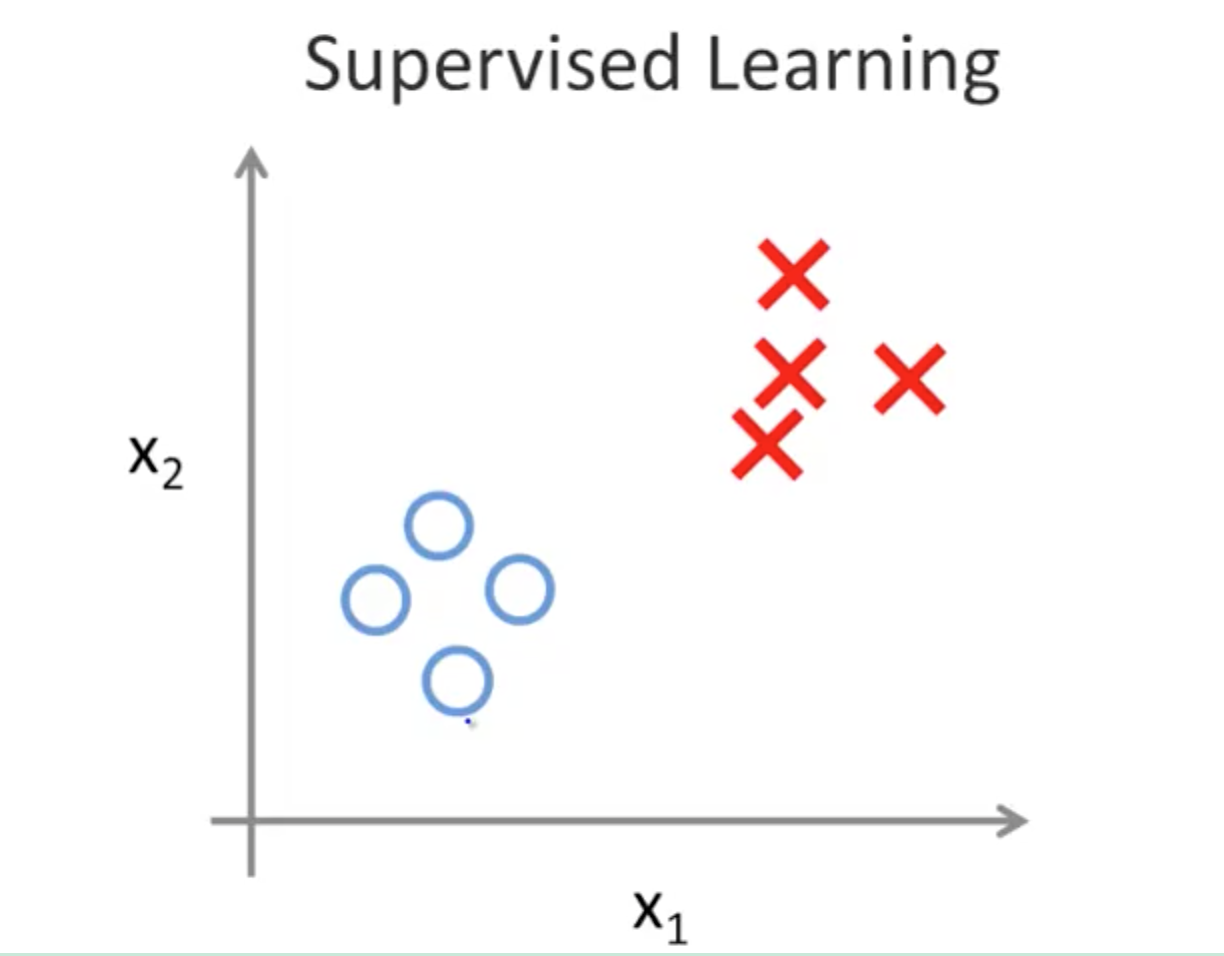### 1a. Classification

• Discrete valued output (0 or 1)
• Example: Breast cancer (malignant and benign)
1. Tumor size
2. Age
• Classify 2 clusters to determine which is more likely

### 1c. Problem 1 (Regression Problem)

You have a large inventory of identical items. You want to predict how many of these items will sell within the next 3 months. 10000 items (continuous value)

### 1d. Problem 2 (Classification Problem)

You’d like a software to examine individual accounts and decide, for each account, if it has been hacked/compromised. 0: not hacked 1: hacked

## 2. Unsupervised Learning

• Making sense of data; patterns we don’t know in advance
• Examples
• Social network analysis
• Market segmentation
• Astronomical data analysis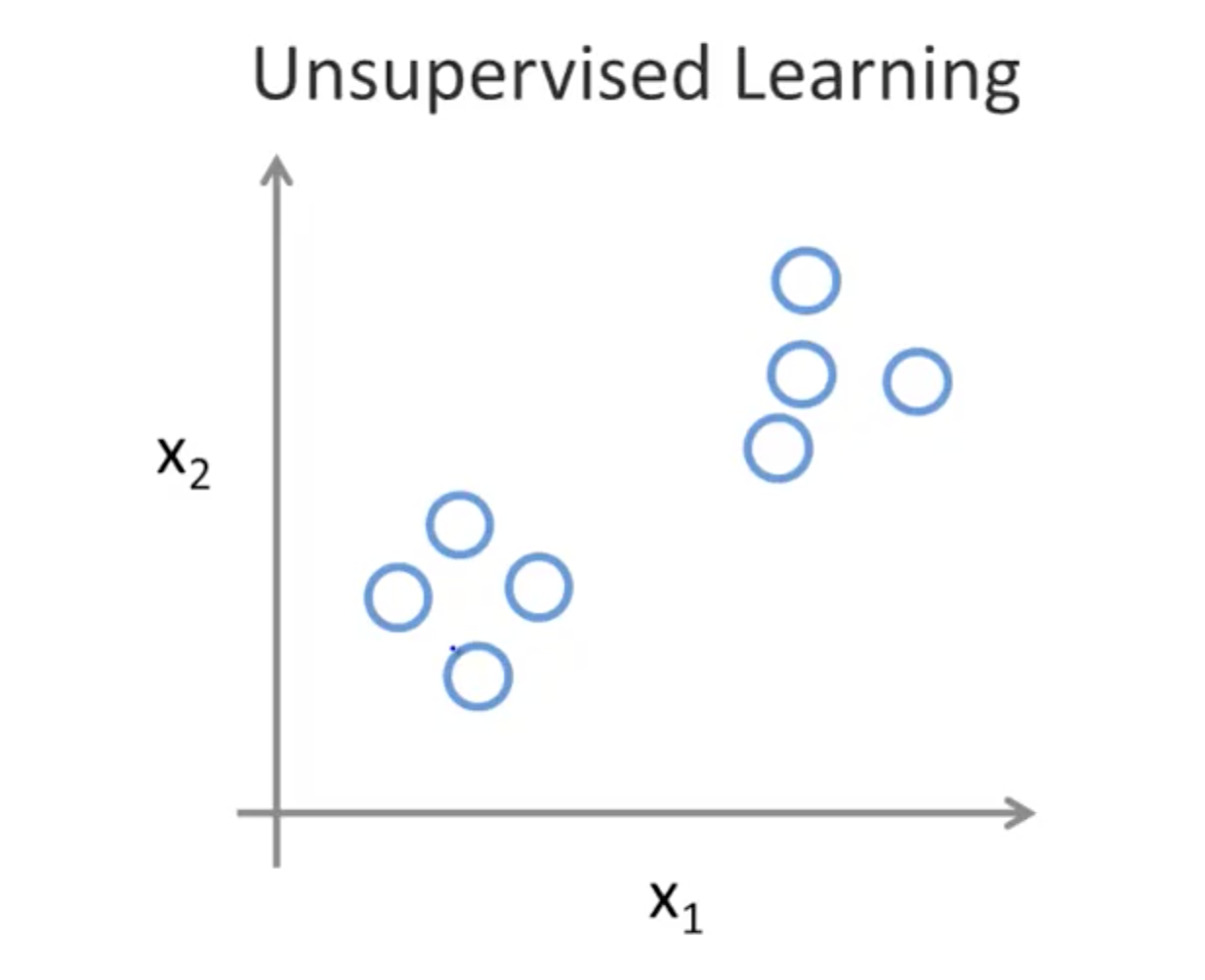### 2a. Cocktail Party Problem

• Give 2 audio recording
• Feed to unsupervised learning algorithm
• Find structure
• Separate audio sources

### 2b. Unsupervised Learning Problems

1. Given a set of news articles found on the web, group them into set of articles about the same story
2. Given a database of customer data, automatically discover market segments and group customers into different market segments

## 3. Model and Cost Function

### 3a. Model Representation

• Supervised learning: given the right answer for each example in the data
• Regression: predict real-valued output
• Classification: predict discrete-valued output
• Training set: original data
• m = number of training examples
• x = input variable/features
• y = output variable/target
• (x, y) = one training example
• (x^(i), y^(i)) = i-th training example
• Representing hypothesis h
• linear regression with one variable (univariate linear regression)
• h(x) = a + bx
• a,b: parameters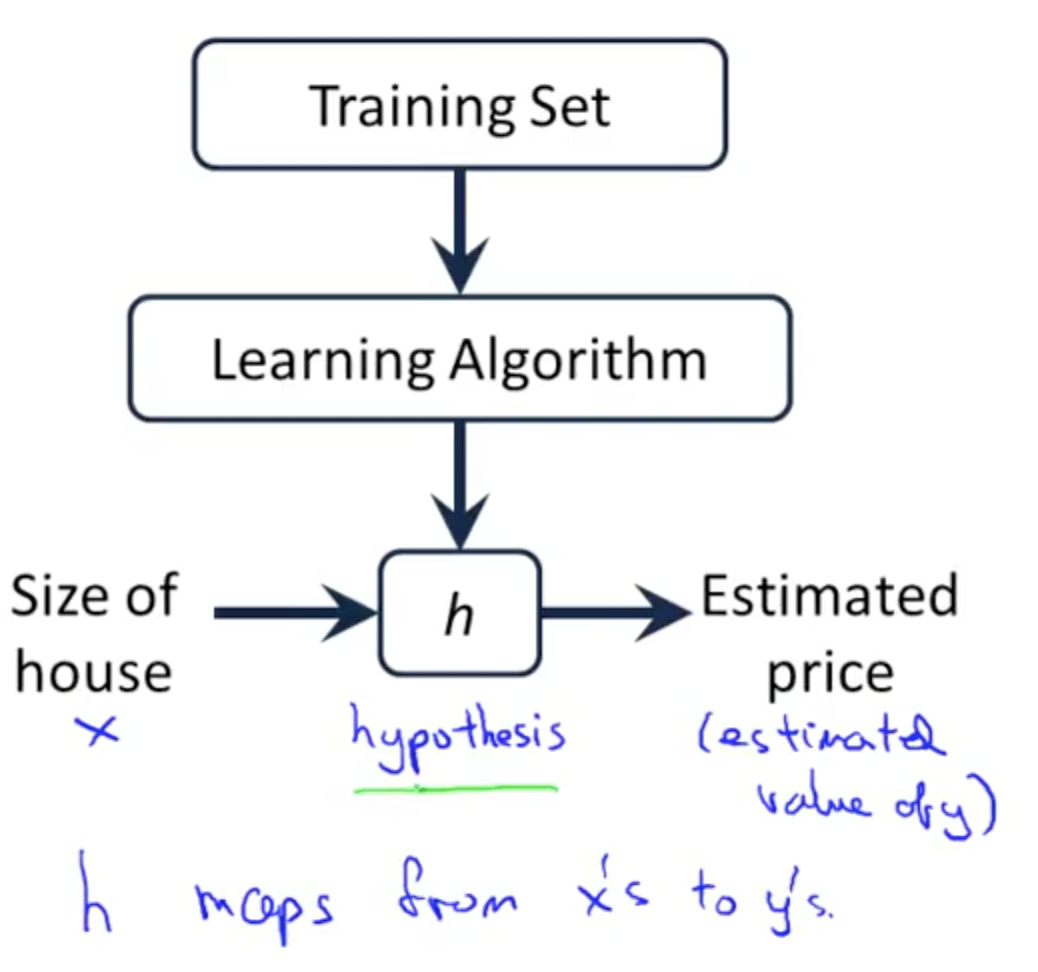### 3b. Cost Function

• Minimise squared error function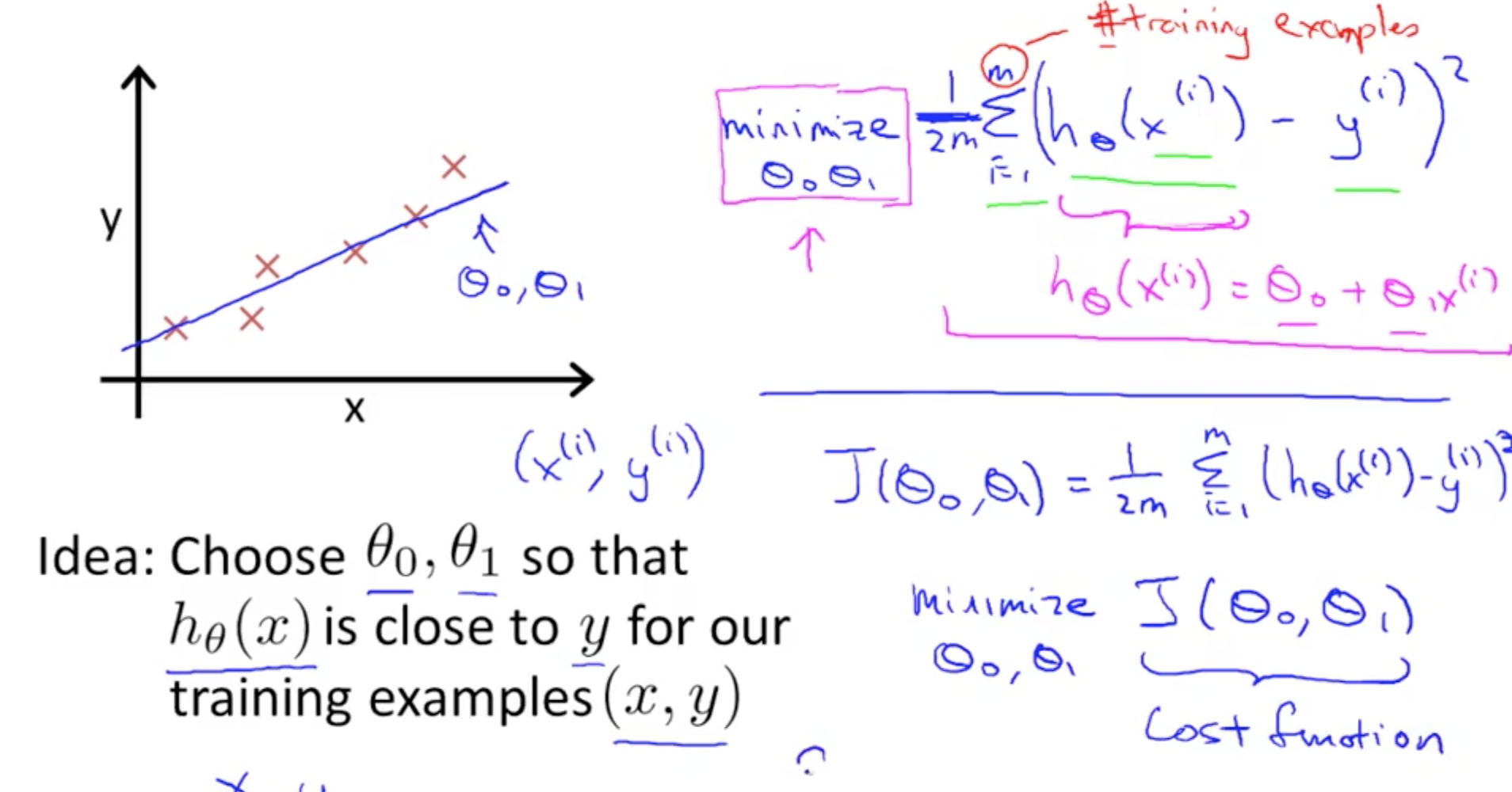### 3c. h(x) and J(theta): 1 Parameter

• Assuming simplified cost function with only theta1
• Minimising J(theta) would fit the data most well### 3d. h(x) and J(theta0, theta1): 2 Parameters

• 3D graph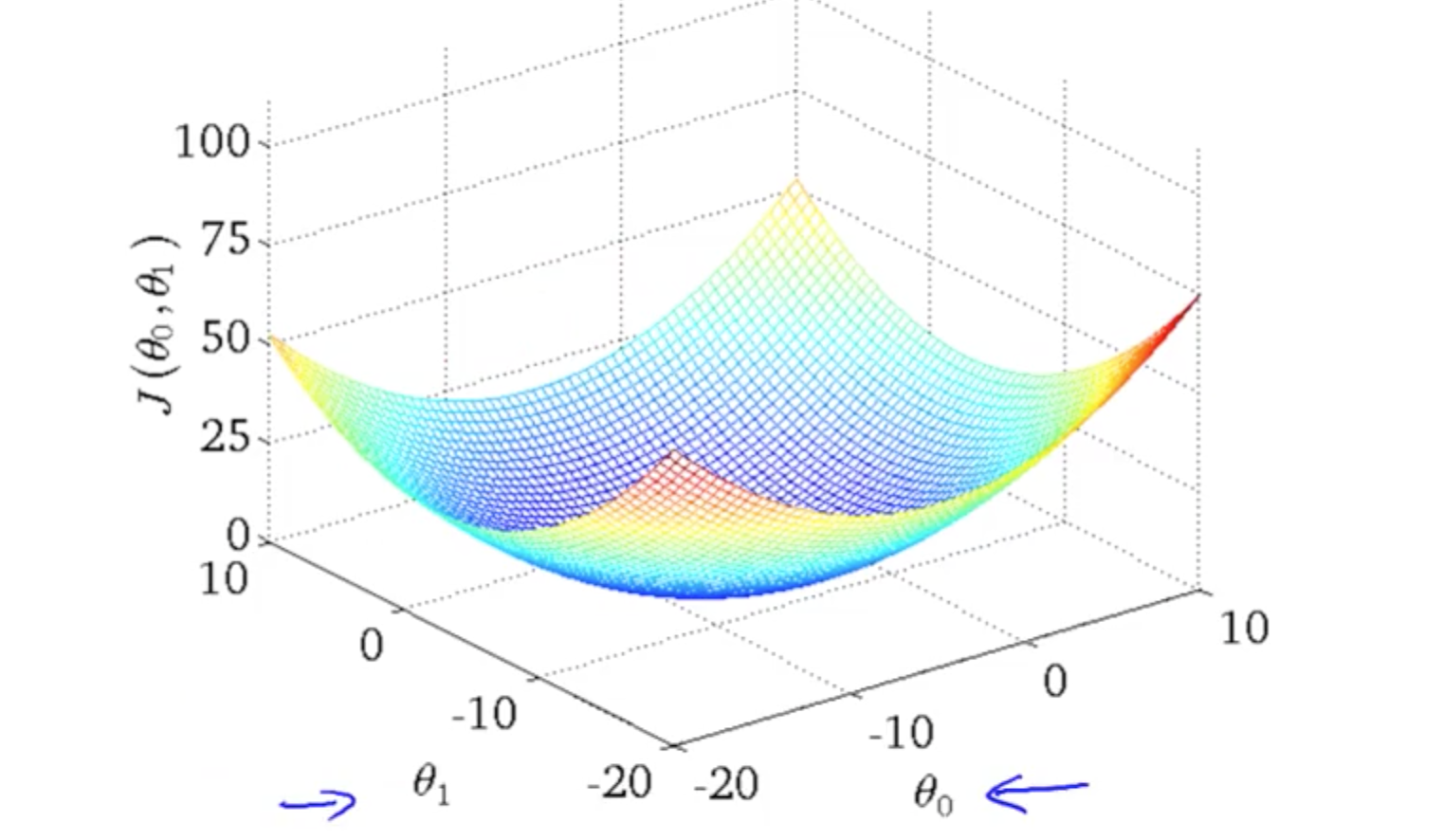• Contour graphs

• Minimum is in the smallest concentric circle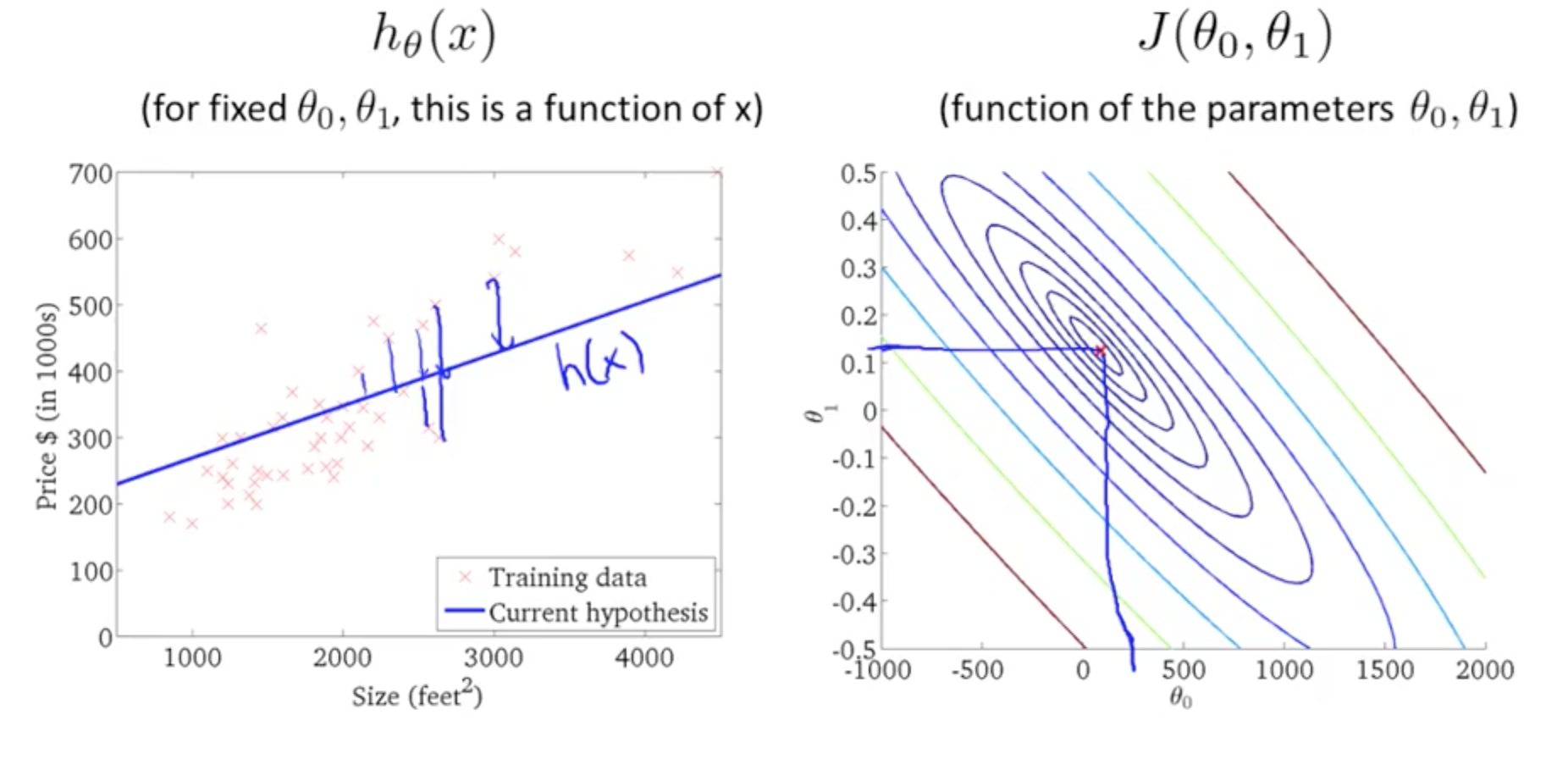## 4. Parameter Learning

• Concept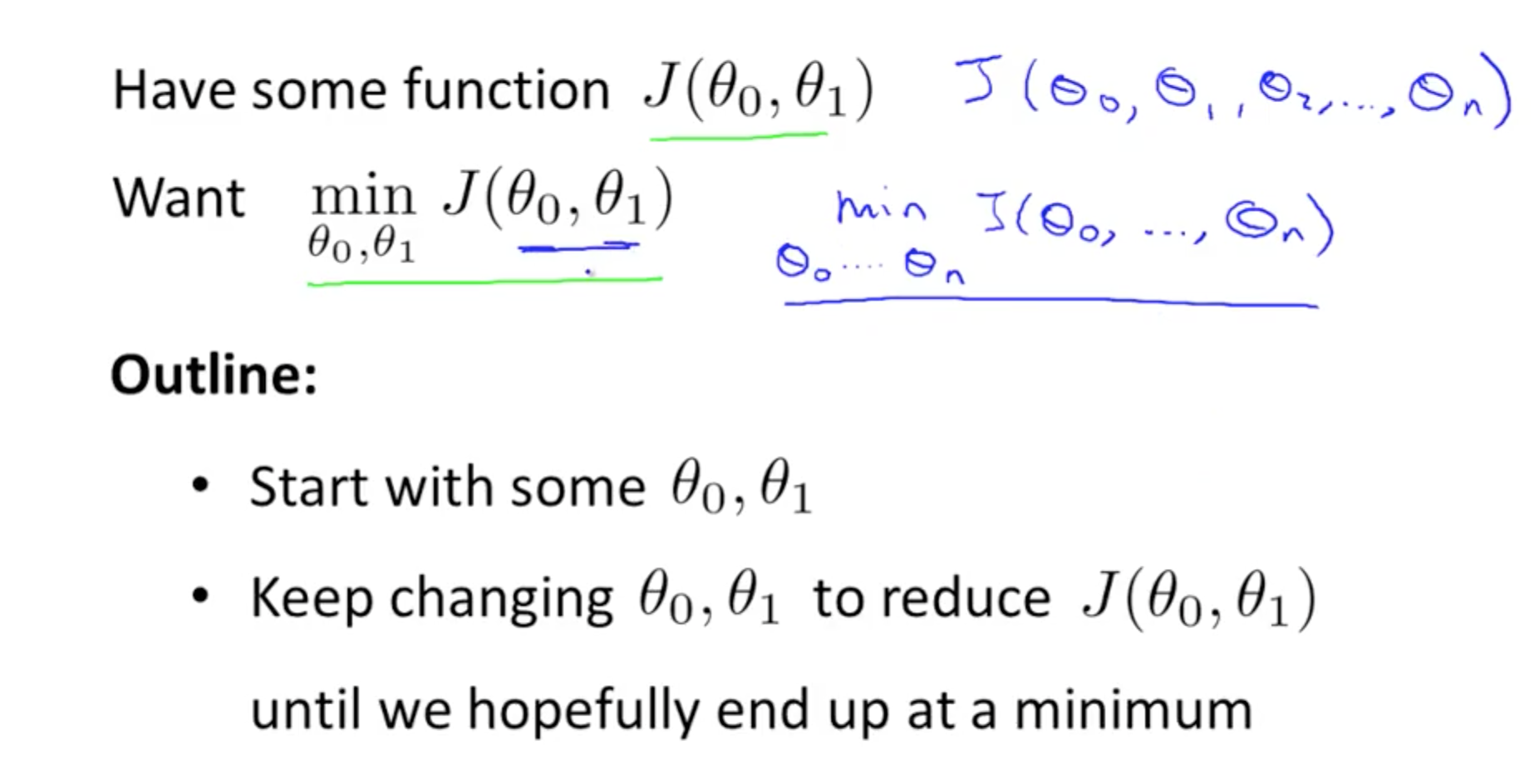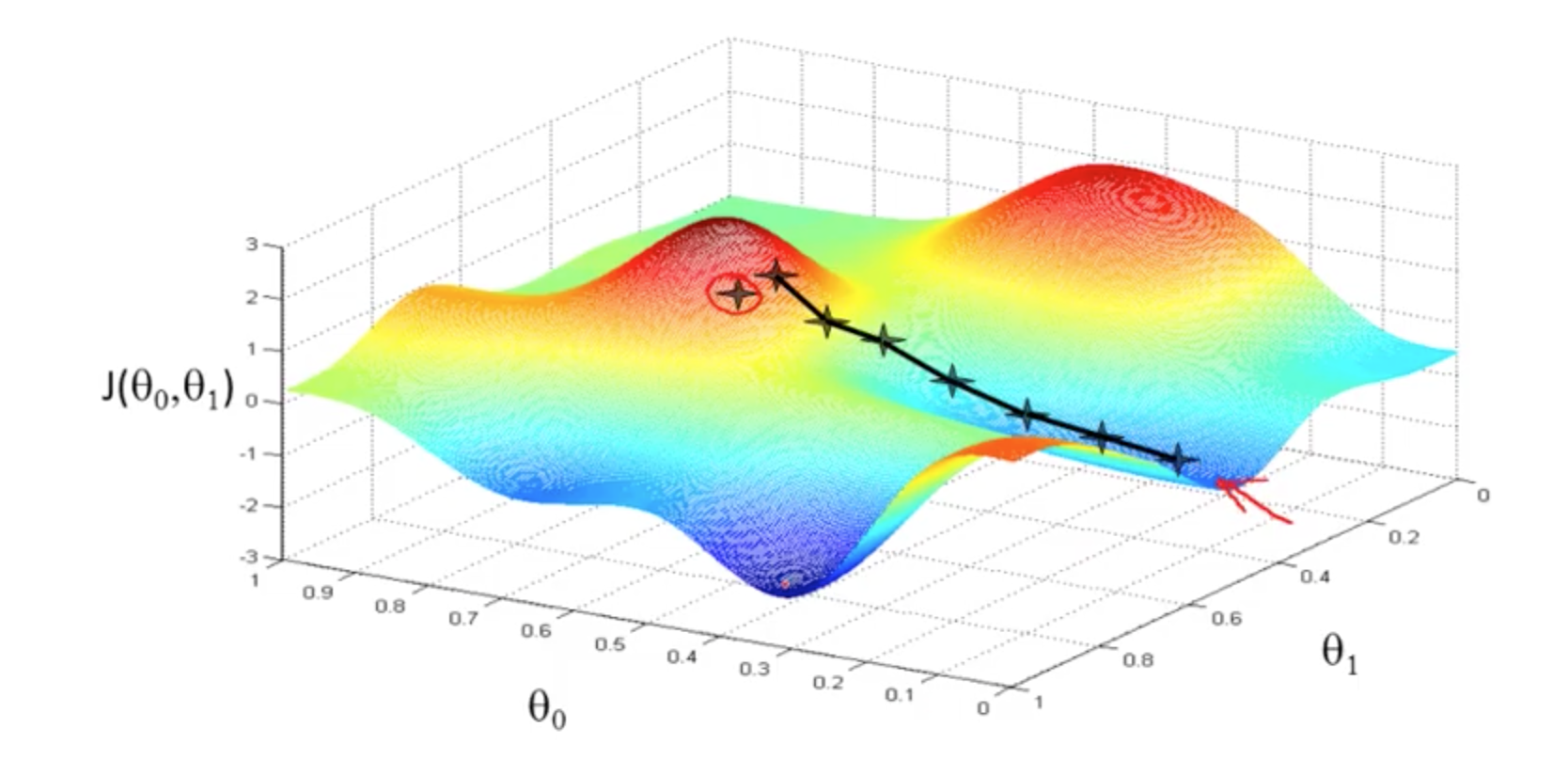• repeat until convergence
• a:= b (this means assignment)
• a = b (truth assertion)
• alpha (number, learning rate)
• derivative: slope of J(theta)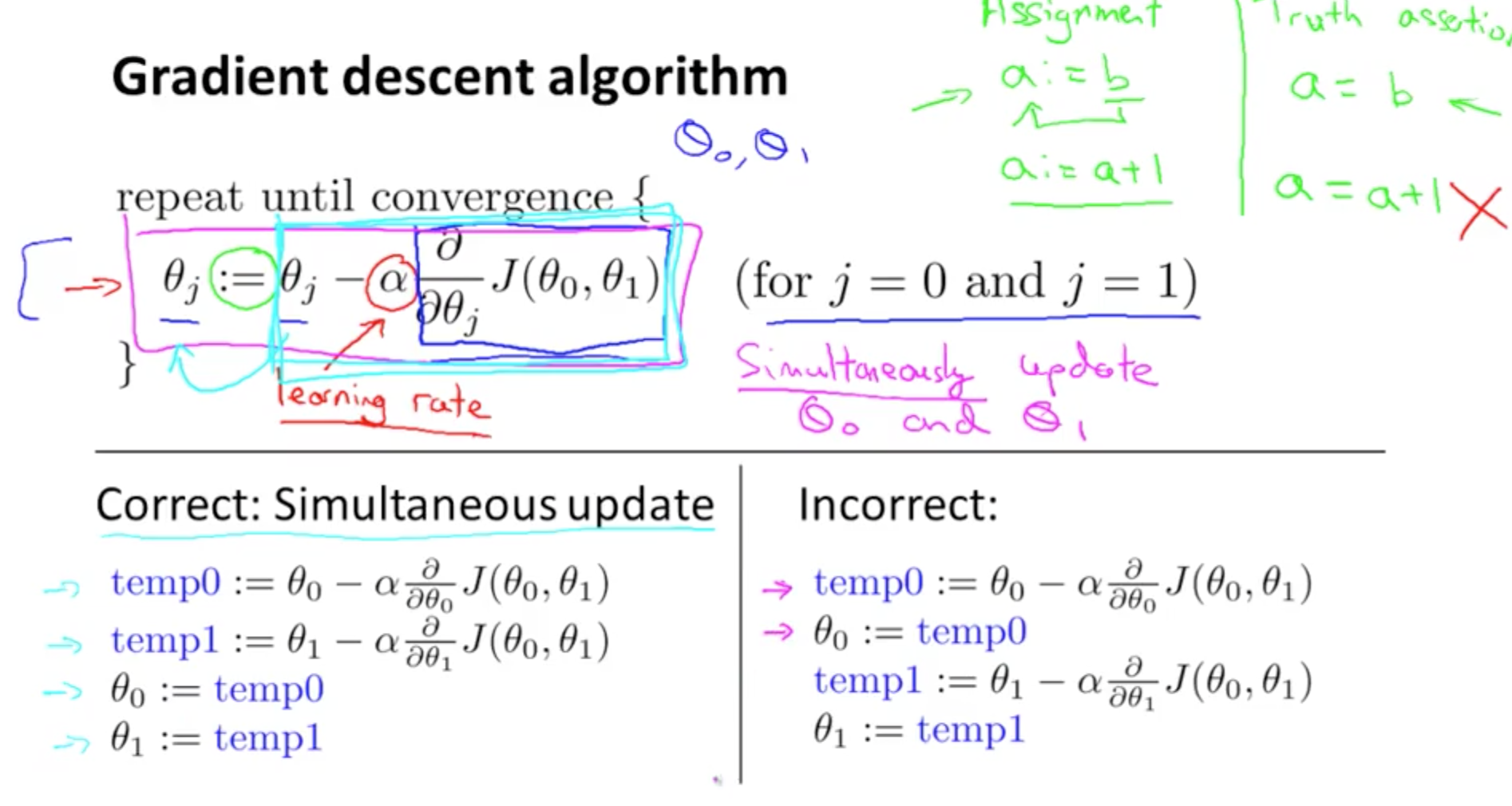• Derivative intuition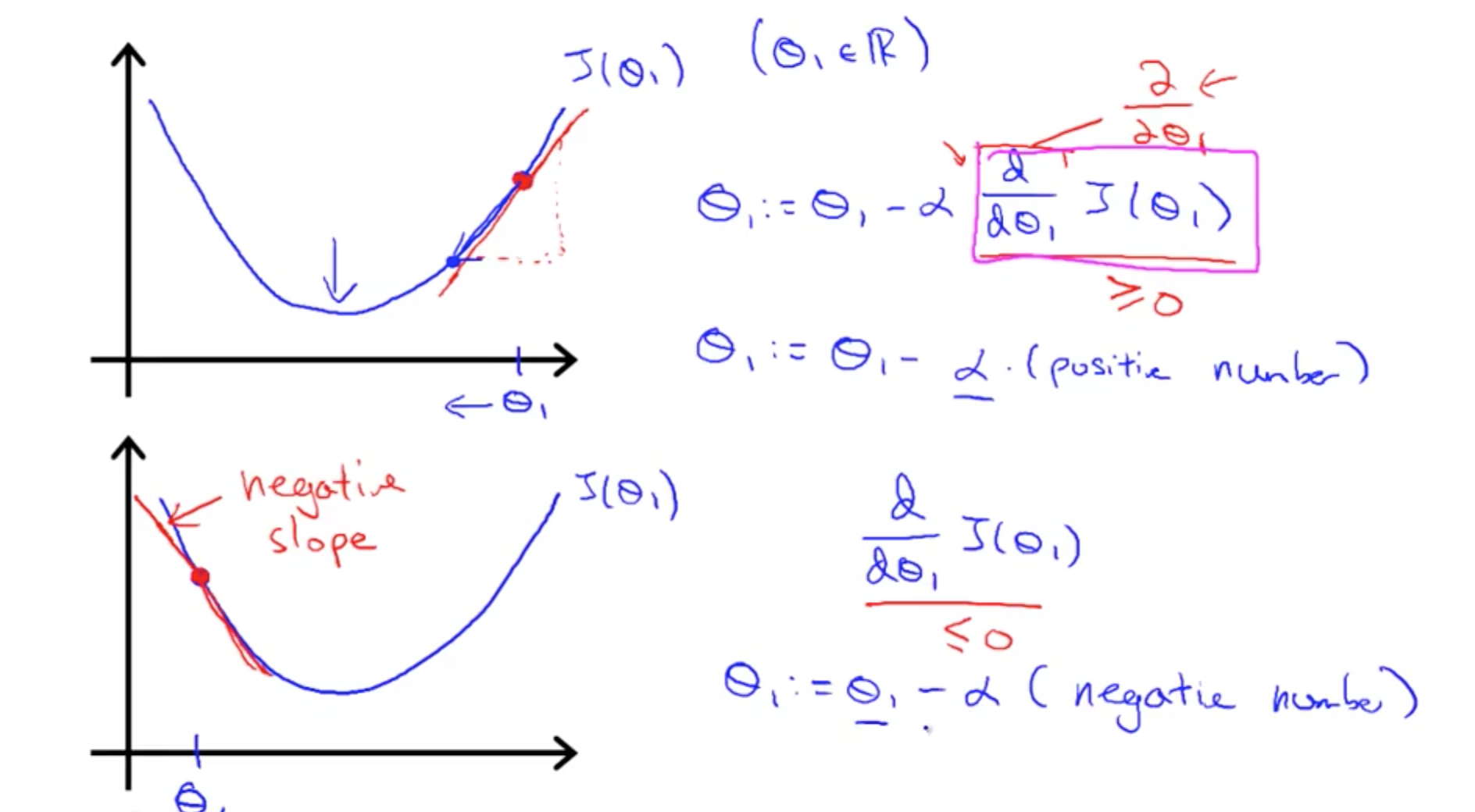• Alpha intuition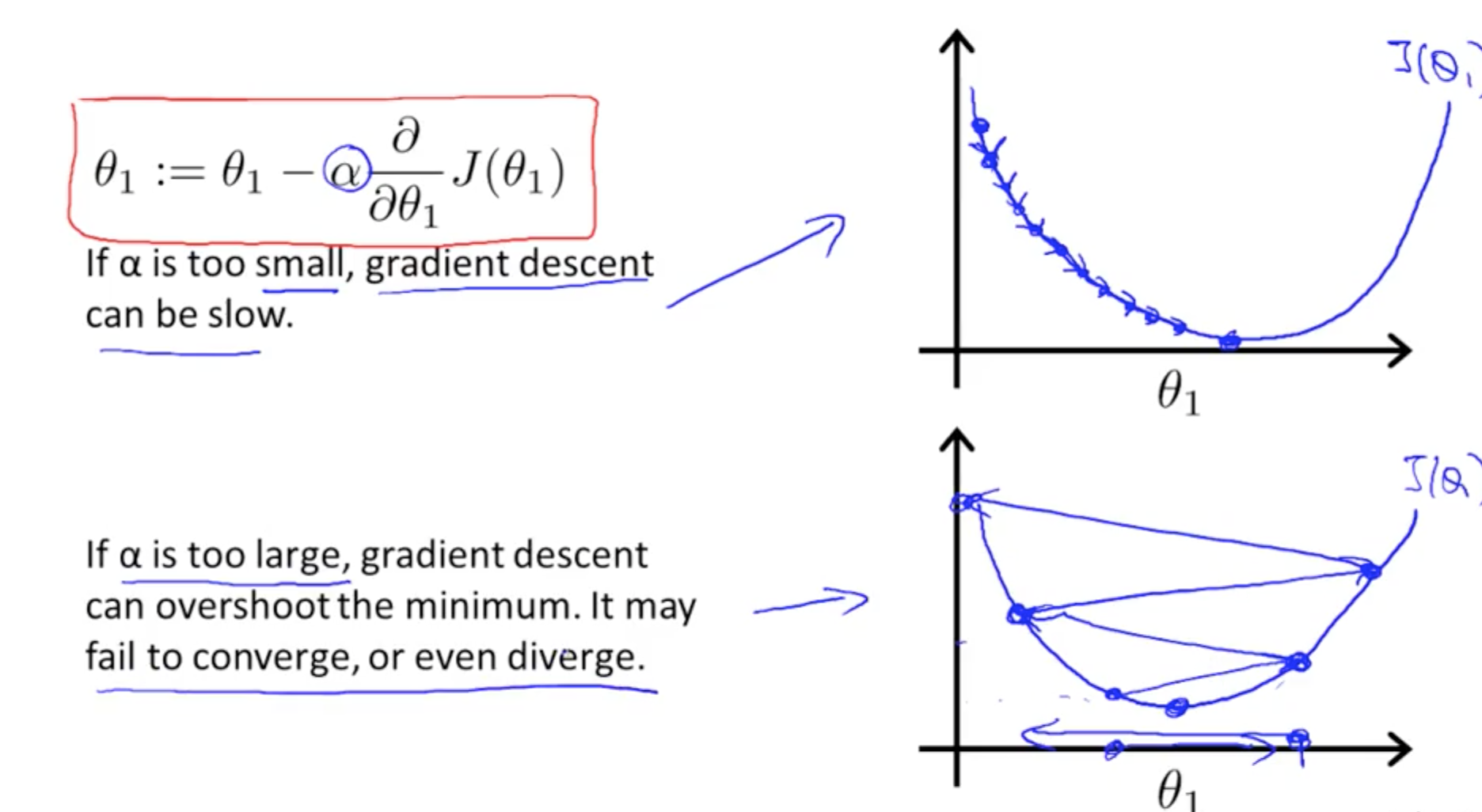• If already at local optima –> derivative = 0
• Theta will not change!
• Steps will be smaller even with alpha fixed
• This is because derivative decreases (slope decreases) nearing local minimum

### 4c. Gradient Descent for Linear Regression

• Apply gradient descent algorithm to linear regression
• For derivative: d (single parameter), delta (multiple derivative, partial differentiation)
• Plug J(theta_0, theta_1) into Gradient Descent’s derivative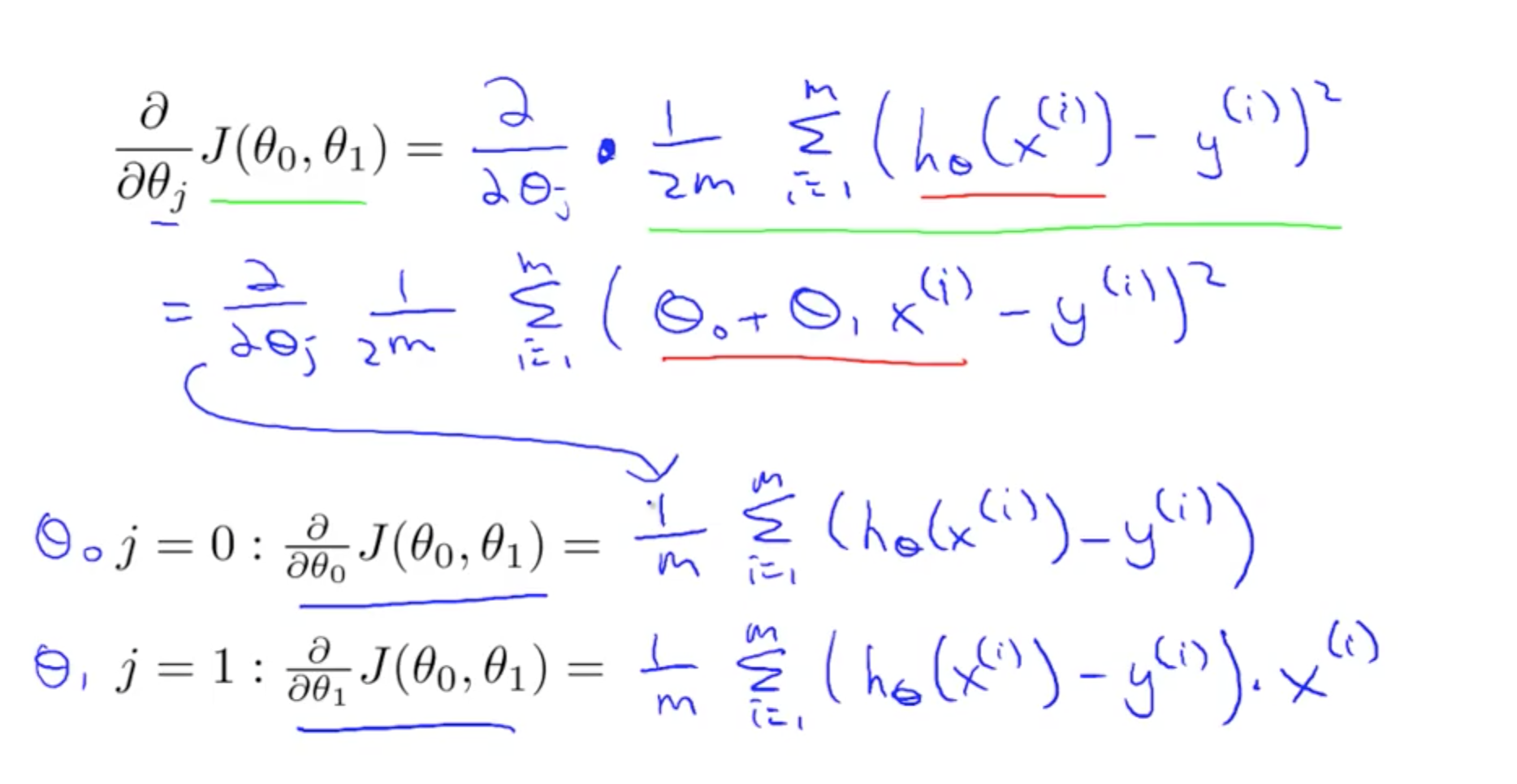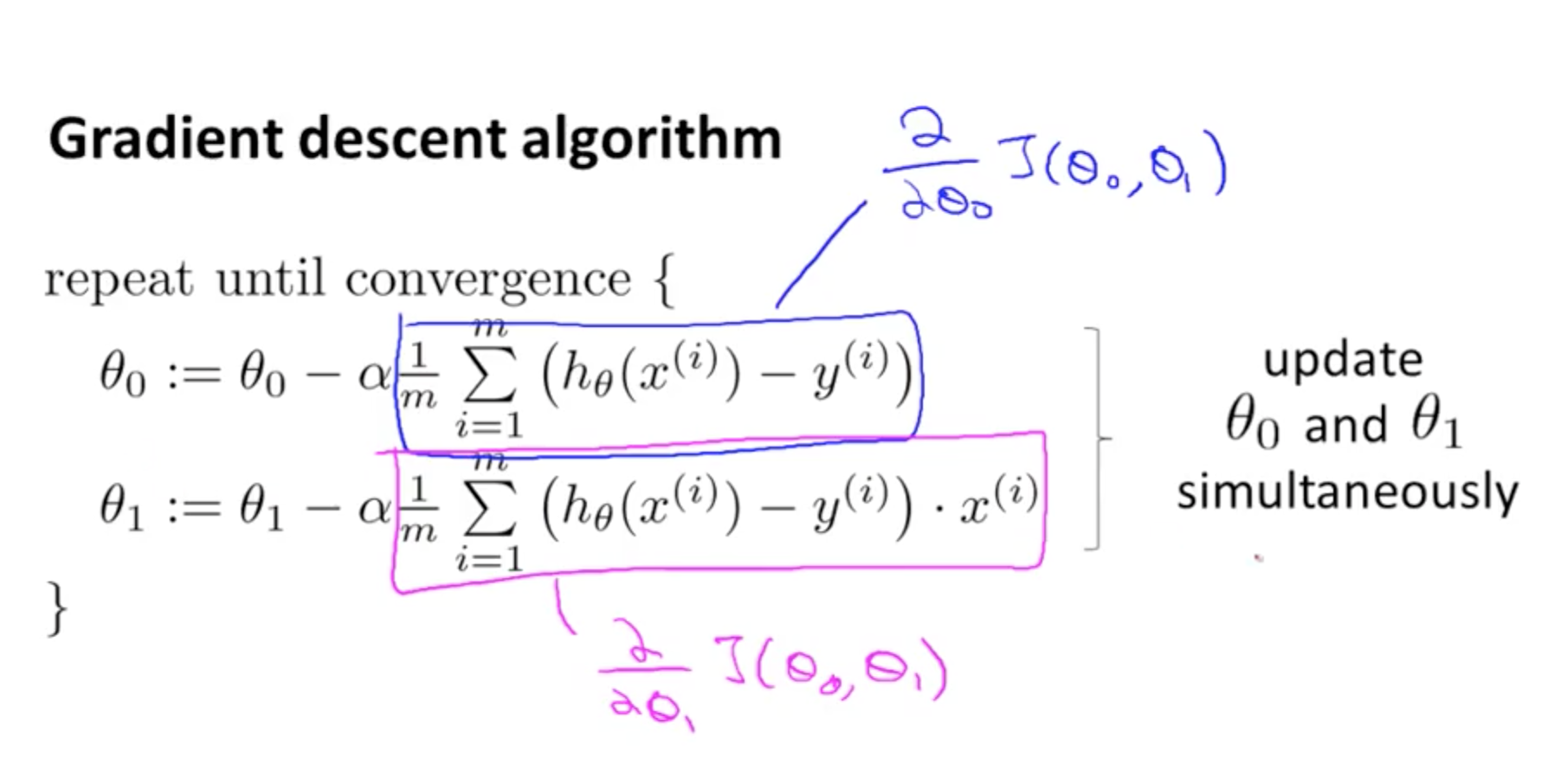• Cost function for linear regression will always be convex function
• One global minimum• Gradient descent for linear regression
• Keep changing parameters to reach global minimum
• This is called “Batch Gradient Descent”
• Each step uses all the training examples (batch)